# Virtual Bolts Connectors – Accurate Results: SOLIDWORKS SIMULATION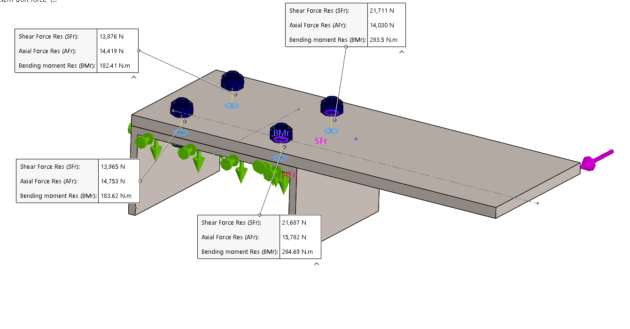In SOLIDWORKS Simulation bolt connectors allows us to define bolts without the need for a physical bolt modeled and meshed in our assembly. We can define preload for these bolts, pitch value, a factor of safety.

As bolts are usually much smaller than the size of the 3D model itself, we would need to define mesh control which will increase the number of elements in our simulation model and take longer to solve.

However, with bolt connectors, we don’t have the physical bolts in the model and no mesh is needed for bolts.

In this article, we want to quickly see how we can check bolts strength using virtual bolt connectors in static analysis and compare the results with theoretical results to check how accurate are these virtual bolt connectors.

The following image shows a 15 * 200 mm rectangular steel bar which is cantilevered to 250 mm steel channel using four tightly fitted bolts at A B C & D and a vertical force of 16Kn is applied at the free end.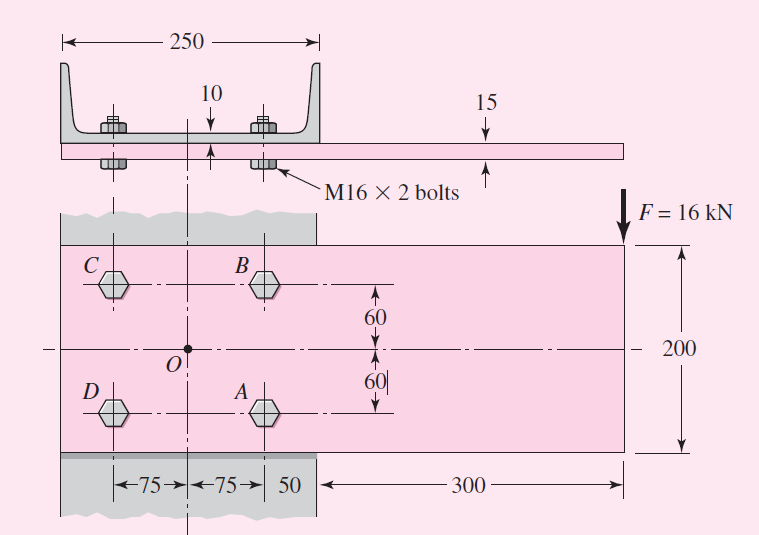From the theoretical approach, we can calculate the strength of bolts by calculating resultant shear forces on each bolt.  Point O, the centroid of the bolt group in Figure is found by symmetry.

If a free-body diagram of the beam were constructed, the shear reaction V would passthrough O and the moment reactions M would be about O. These reactions are

V = 16 KN and M = 16(425) = 6800 N-m.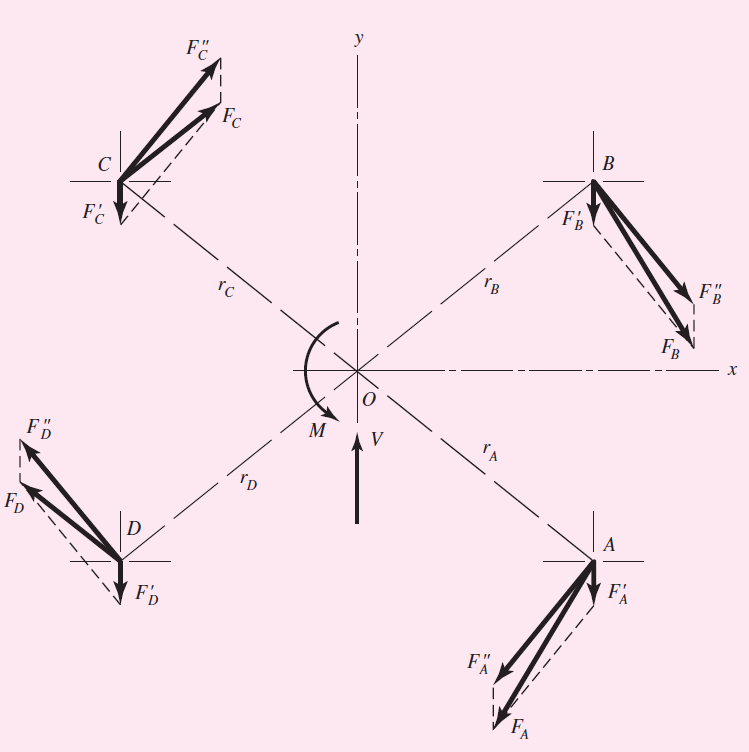The bolt group has been drawn to a larger scale and the reactions are shown in the figure. The distance from the centroid to the center of each bolt is,

r =  = 96 mm.

The primary shear load per bolt is

F’ = V/n = 16/4 = 4 kN

Since the secondary shear forces are equal,

F’’ = Mr/4r2 = M/4r = 6800/4(96.0) = 17.7 kN

The primary and secondary shear forces are plotted to scale in Figure and resultants obtained by parallelogram law,

FA = FB = 21.0 kN

FC = FD = 14.8 kN

In SOLIDWORKS SIMULATION we can get similar results by making use of bolt connectors without having to physically model the bolts. Following is the simple step to define the virtual bolts by adding all the parameters of the bolts.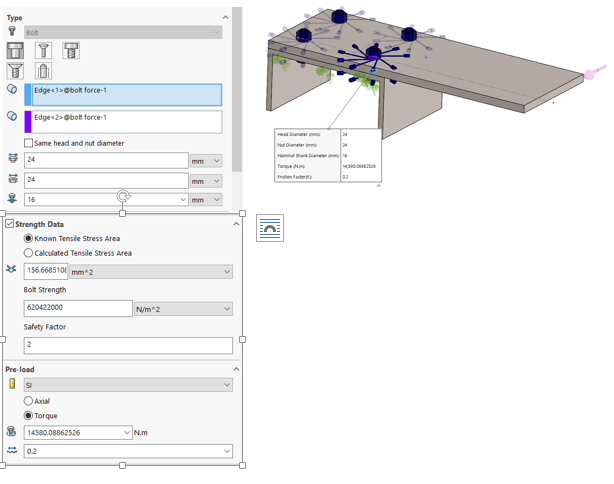From Postprocessing in SOLIDWORKS SIMULATION we can find the resultant axial and shear force acting on these bolt connectors which is shown below,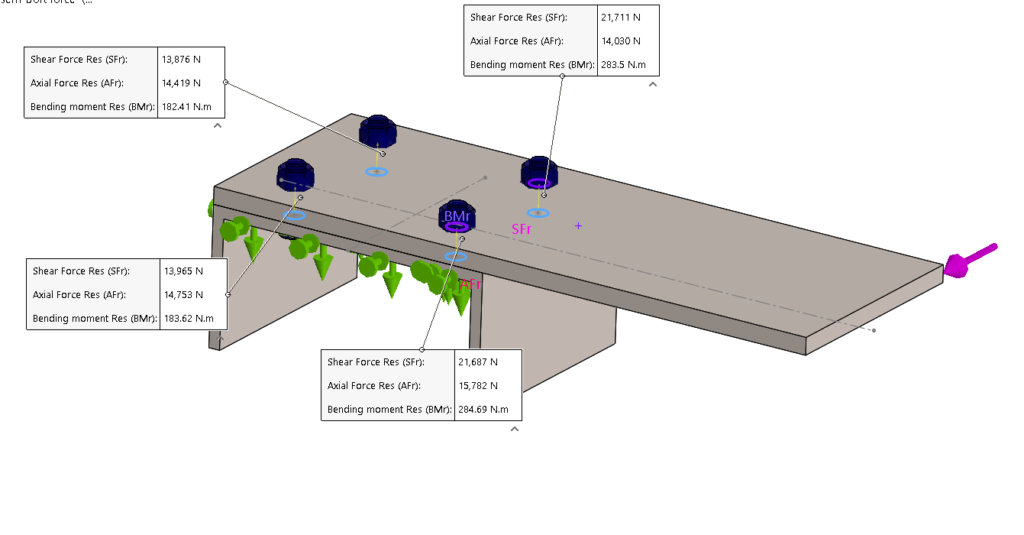Following is the comparison list of results for the theoretical approach and FEA approach using SOLIDWORKS SIMULATION.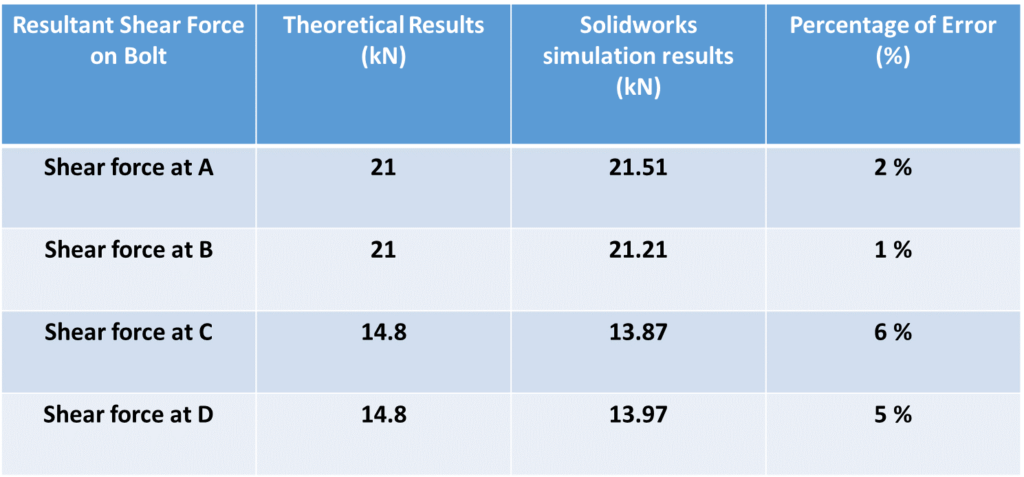Virtual bolt connectors in SOLIDWORKS SIMULATION not only save the time of physically modeling, meshing of bolts but also yield accurate results.

We Urge You To Call Us For Any Doubts & Clarifications That You May Have. We Are Eager to Talk To You

Call Us: +91 7406663589

### For SOLIDWORKS Product Enquiry Contact:

##### AUTHOR: BeaconIndia(2 votes, average: 5.00 out of 5)Loading...